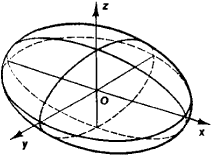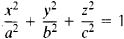# ellipsoid

(redirected from Franz Schweigger-Seidel)
Also found in: Dictionary, Thesaurus, Medical.

## ellipsoid

a geometric surface, symmetrical about the three coordinate axes, whose plane sections are ellipses or circles. Standard equation: x2/a2 + y2/b2 + z2/c2 = 1, where ?a, ?b, ?c are the intercepts on the x-, y-, and z- axes
Collins Discovery Encyclopedia, 1st edition © HarperCollins Publishers 2005

## ellipsoid

(i-lip -soid) A surface or solid whose plane sections are circles or ellipses. An ellipse rotated about its major or minor axis is a particular type of ellipsoid, called a prolate spheroid (major axis) or an oblate spheroid (minor axis).
Collins Dictionary of Astronomy © Market House Books Ltd, 2006
The following article is from The Great Soviet Encyclopedia (1979). It might be outdated or ideologically biased.

## Ellipsoid

a closed central quadric surface. An ellipsoid has a center of symmetry O (see Figure 1) and three axes of symmetry, which are called the axes of the ellipsoid. The plane sections ofFigure 1

ellipsoids are ellipses; in particular, one can always find a plane section that is a circle. In a suitable coordinate system the equation of an ellipsoid has the form